ENGR338 Lab Spring 2021
Lab 5: The Inverter
Name: Audra Benally

Email: albenally1@fortlewis.edu

1. Title: The Inverter

2. Introduction: In this lab, we built an inverter in schematic and layout view. This inverter had a PMOS with a width of 20 and an NMOS with a width of 10. Next we used this first inverter to make a larger inverter using the multiplier function in ElectricVLSI. The layout for the larger inverter had a PMOS of width 100 and an NMOS of width 50. The two inverters were connected to different sized capacitors and simulated to test their driving capabilities. New built-in simulation tools were introduced in this lab as well.

3. Materials and Methods:
Materials:

- Computer
- LTSpice Software
- ElectricVLSI Software
Methods:
For this lab we created two different versions of a PMOS/NMOS inverter. The first inverter was a 20/10 inverter with 20/2 PMOS and 10/2 NMOS components. The inverter was built in schematic view as seen in figure 1 first, then in layout view which can be seen in figure 3. After schematic view was made, code was added and simulated to show the simulation in figure 2. Then, a new inverter was made using the schematic of the first inverter. A 5x multiplier was added through tools that turned the old components into a 100/10 PMOS and a 50/10 NMOS. In the layout view the PMOS and the NMOS were both duplicated and lengthened to what is now shown in figure 5. Both inverters were simulated with three different capacitors. The first had a capacitance of 100fF, the second was 1pF, and the third was 10pF. The simulations for the first 20/10 inverter is seen in figure 6 while the simulation for the 100/50 inverter can be seen in figure 7. For the last task, different simulation tools were used to test the two inverters. Figure 8 shows the ALS simulation tool while figure 9 shows the same simulation with the IRSIM simulation tool.

4. Results:Figure 1. Inverter schematic for the first 20/10 inverter.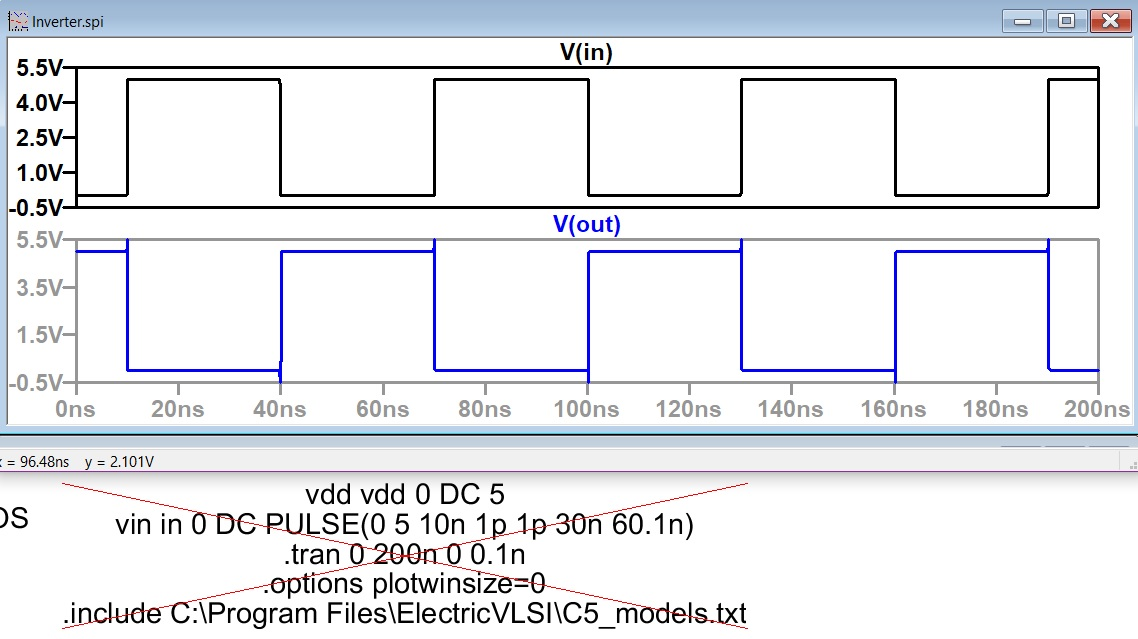Figure 2. Modified spice code to run a transient simulation using a PULSE() Vinput.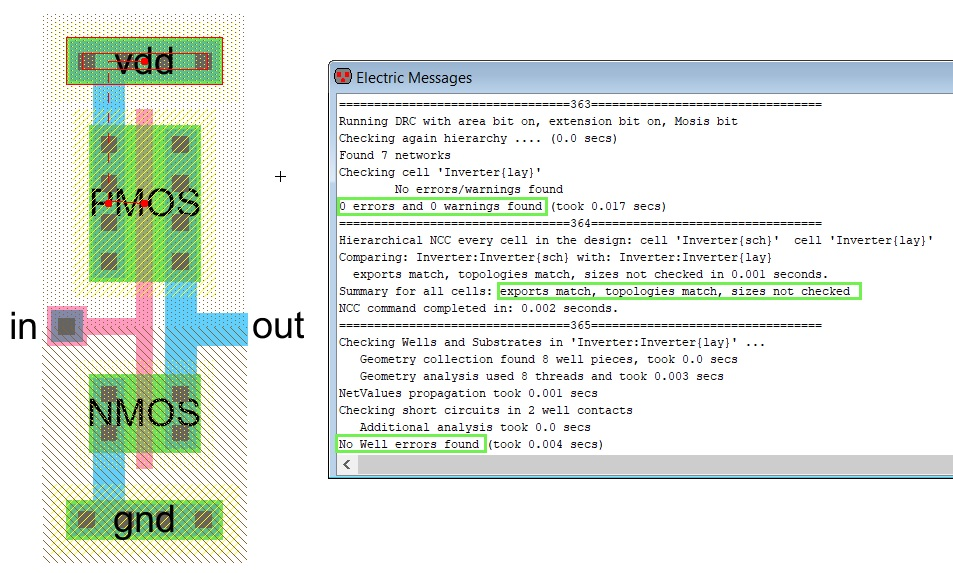Figure 3. 20/10 Inverter layout with DRC, NCC, and well check results highlighted.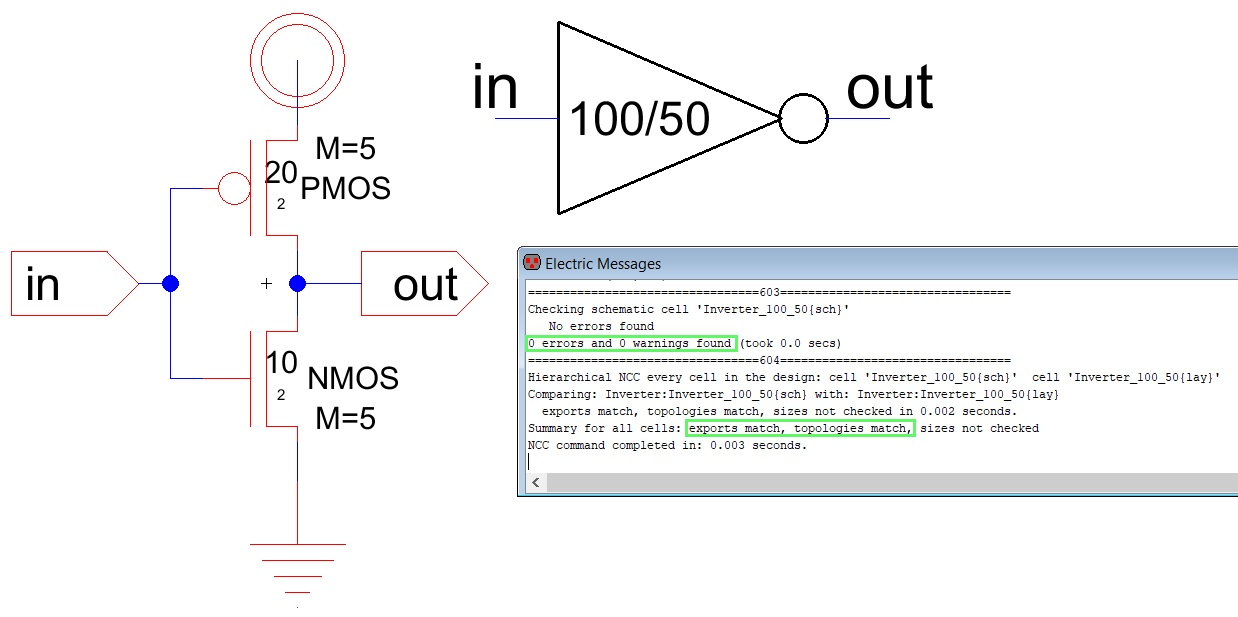Figure 4. 100/50 Inverter schematic made from 20/10 schematic with M=5.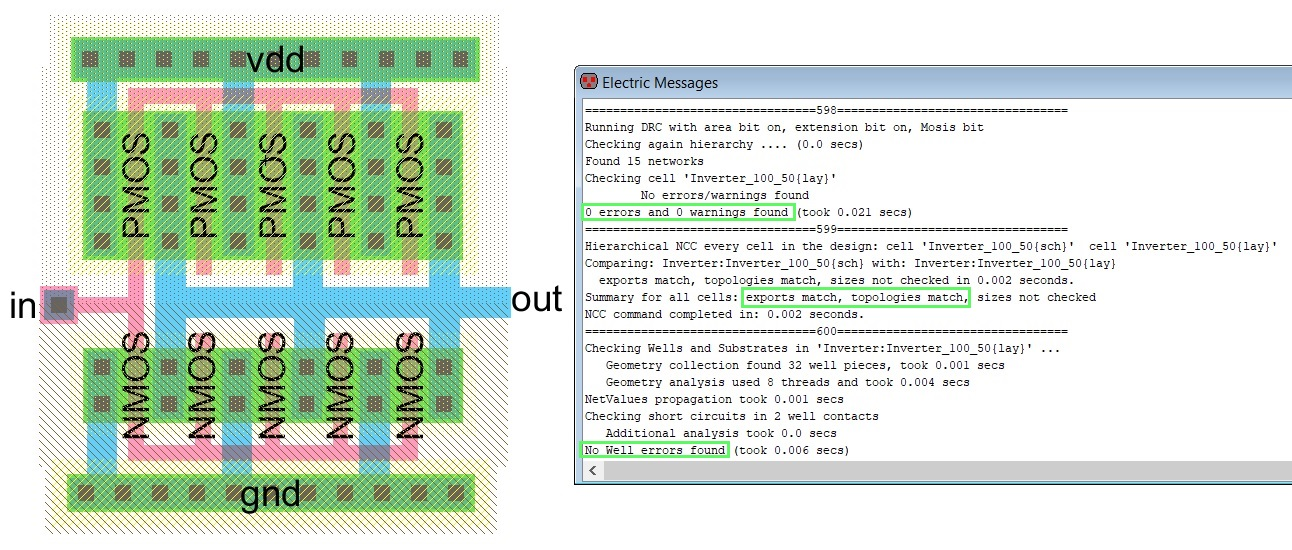Figure 5. 100/50 Inverter layout with DRC, NCC, and well check results highlighted.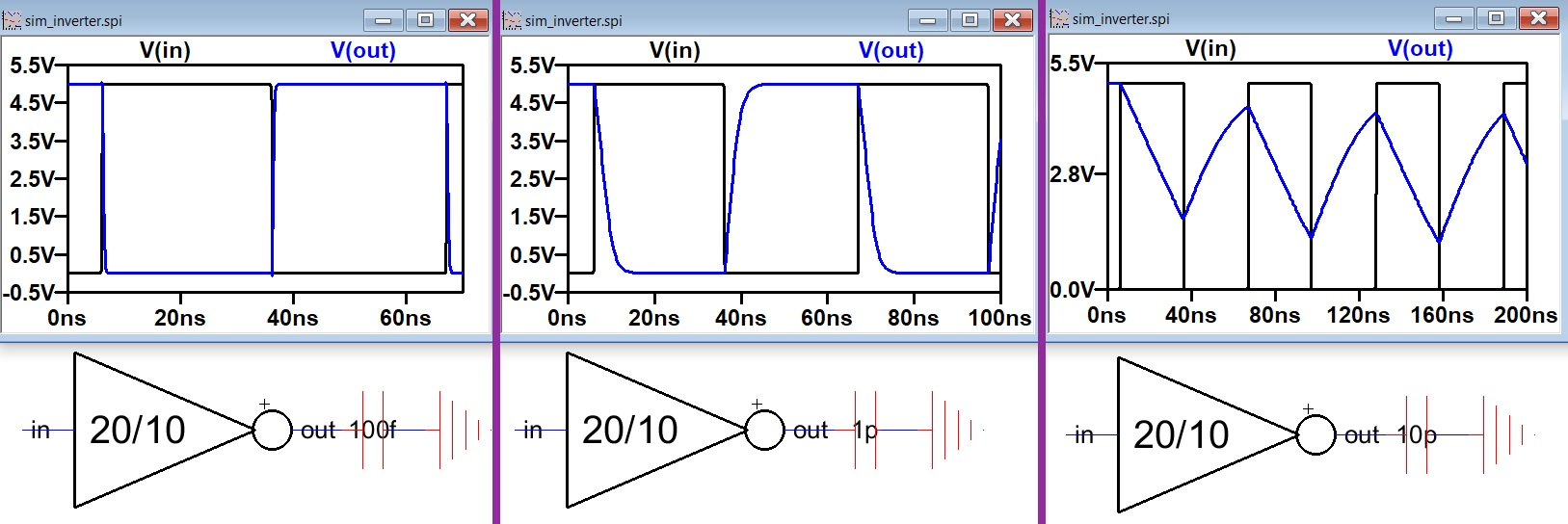Figure 6. 20/10 Inverter with different capacitors and the resulting Vin/Vout simulations. The simulations show the inverter's driving capabilities.Figure 7. 100/50 Inverter connected to the same capacitors as the 20/10 inverter. The resulting simulations are grouped with their schematics. The simulations show the inverter's driving capabilities.Figure 8. Simulation using the built-in ALS simulation tool.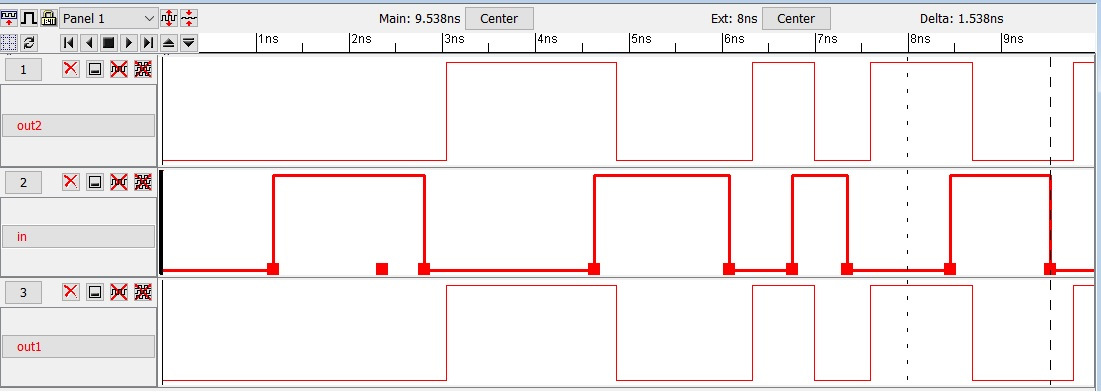Figure 9. Simulation using IRSIM simulation tool.

5. Discussion